# Resource Title search

#### Text Search of NRICH resource titles

##### Or find resource begining with:### Bio Graphs

##### Age 14 to 16 Challenge Level:

What biological growth processes can you fit to these graphs?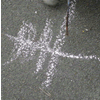### Children's Mathematical Graphics: Understanding the Key Concept

##### Age 5 to 7

In this article for teachers, Elizabeth Carruthers and Maulfry Worthington explore the differences between 'recording mathematics' and 'representing mathematical thinking'.### Coordinate Geometry and Graphs

##### Age 16 to 18### Core Maths - Graphs

##### Age 16 to 18 Challenge Level:

These resources are suitable for teaching about graphs in Core Maths### Factors and Multiples Graphs

##### Age 14 to 18 Challenge Level:

Explore creating 'factors and multiples' graphs such that no lines joining the numbers cross### Functions & Graphs: Age 11-14

Working on these problems will help students develop a better understanding of functions and graphs.### Functions & Graphs: Age 14-16

Working on these problems will help students develop a better understanding of functions and graphs.### Functions and Graphs - Short Problems

##### Age 11 to 16

A collection of short problems on straight line graphs.### Functions and Graphs - Stage 3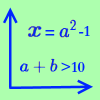### Functions and Graphs - Stage 3

##### Age 11 to 14

Working on these problems will help you develop a better understanding of functions and graphs.### Functions and Graphs - Stage 3

Working on these problems will help you develop a better understanding of functions and graphs.### Functions and Graphs - Stage 4

Working on these problems will help you develop a better understanding of functions and graphs.### Graph Area

##### Age 11 to 14 Short Challenge Level:

Can you find the area between this graph and the x-axis, between x=3 and x=7?### Graph Triangles

##### Age 14 to 16 Short Challenge Level:

Use the information about the triangles on this graph to find the coordinates of the point where they touch.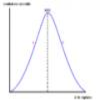### Graphic Biology

##### Age 16 to 18 Challenge Level:

Several graphs of the sort occurring commonly in biology are given. How many processes can you map to each graph?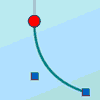### Graphical Interpretation

##### Age 14 to 16 Challenge Level:

This set of resources for teachers offers interactive environments to support work on graphical interpretation at Key Stage 4.### Graphical Triangle

##### Age 14 to 16 Short Challenge Level:

What is the area of the triangle formed by these three lines?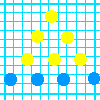### Graphing Number Patterns

##### Age 7 to 11 Challenge Level:

Does a graph of the triangular numbers cross a graph of the six times table? If so, where? Will a graph of the square numbers cross the times table too?### Graphs

##### Age 11 to 16 Challenge Level:

The graphs collection of STEM resources### Graphs

Graph related STEM resources### Graphs and Networks

##### Age 14 to 18 Challenge Level:

Be playful with graphs and networks, and see what theorems you can discover!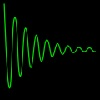### Graphs Make Sense of Data

##### Age 11 to 16

Graphs are a crucial tool in dealing with the data that science generates.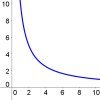### Graphs of Changing Areas

##### Age 16 to 18 Challenge Level:

Use graphs to gain insights into an area and perimeter problem, or use your knowledge of area and perimeter to gain insights into the graphs...### Guessing the Graph

##### Age 14 to 16 Challenge Level:

Can you suggest a curve to fit some experimental data? Can you work out where the data might have come from?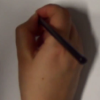### How to Sketch Graphs

##### Age 16 to 18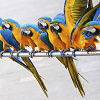### Lining up for Line Graphs

##### Age 11 to 14 Challenge Level:### Name That Graph

##### Age 16 to 18 Challenge Level:

How can you work out the equation of a parabola just by looking at key features of its graph?### Name That Graph Again

##### Age 16 to 18 Challenge Level:

If you know some information about a parabola, can you work out its equation?### Numbered Graph

##### Age 16 to 18 Challenge Level:

A weekly challenge: these are shorter problems aimed at Post-16 students or enthusiastic younger students.### Plotting Graphs

##### Age 16 to 18 Challenge Level:

You can get a great feel for functions by sketching their graphs or using graph plotting software...### Public Key Cryptography

##### Age 16 to 18

An introduction to the ideas of public key cryptography using small numbers to explain the process. In practice the numbers used are too large to factorise in a reasonable time.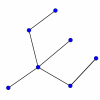### Simply Graphs

##### Age 16 to 18 Challenge Level:

Look for the common features in these graphs. Which graphs belong together?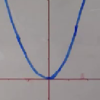### Sketching Graphs - Transformations

##### Age 16 to 18 Challenge Level:

If you can sketch y=f(x), there are several related functions you can also sketch...### Some Circuits in Graph or Network Theory

##### Age 14 to 18

Eulerian and Hamiltonian circuits are defined with some simple examples and a couple of puzzles to illustrate Hamiltonian circuits.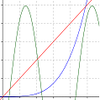### Straight Line Graphs

##### Age 11 to 14

These problems will require you to consider the gradients, intercepts and equations of straight line graphs.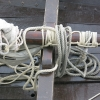### Tangled Trig Graphs

##### Age 16 to 18 Challenge Level:

Can you work out the equations of the trig graphs I used to make my pattern?### The Knapsack Problem and Public Key Cryptography

##### Age 16 to 18

An example of a simple Public Key code, called the Knapsack Code is described in this article, alongside some information on its origins. A knowledge of modular arithmetic is useful.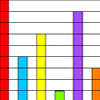### The Pet Graph

##### Age 7 to 11 Challenge Level:

Tim's class collected data about all their pets. Can you put the animal names under each column in the block graph using the information?### Tree Graphs

##### Age 16 to 18 Challenge Level:

A connected graph is a graph in which we can get from any vertex to any other by travelling along the edges. A tree is a connected graph with no closed circuits (or loops. Prove that every tree has. . . .### Useful Graphing Tools

A collection of useful graphing resources to support work on NRICH problems.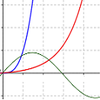### Using Graphs

##### Age 11 to 14

These problems will require you to consider practical contexts in which graphs can be used.### What's That Graph?

##### Age 14 to 16 Challenge Level:

Can you work out which processes are represented by the graphs?### Whose Line Graph Is it Anyway?

##### Age 16 to 18 Challenge Level:

Which line graph, equations and physical processes go together?